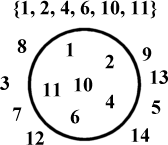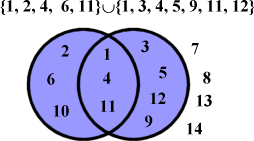# DAY 17. Character Sets

novice intermediate advanced expert

Yesterday we looked at character strings, which are complex data objects based upon characters. Character sets are also complex data objects based upon characters that differ from strings in a very key way: with character strings we care about, nor do we even depend upon the ordering of the characters within the string. With a character set we only care whether a character is in the set or not.

## Sets and Set Theory

A set is any collection of items with three properties.
1. Each member of the set is unique, there can never be more than one of the same set member.
2. The members of a set are unordered.
3. An element can either be a member to a set or not, there are no in-betweens.
Notationally, a set is indicated using braces. The elements can be defined as a list, descriptive sentence, or equation:

{ 0, x, ö, 9, 12, A }
The set of the letters 'x', 'ö', 'A', and the integers '0', '9', '12'.

{ all positive small integers }
The set 1, 3, 5, 7, ...

{ x | x! <= 120 }
The set 1, 2, 6, 24, 120

If a set has no members, it is called the empty set and is denoted Æ, which must be at least just a little annoying to the Swedes.

### Things to do to Your Sets

There are some fundamental set operations defined. To apprehend them fully, we will graphically represent a set as the set members enclosed in circles. E.g.### Complement

The complement of a set S is written S', and is the set of all elements not in the set. Thus, S' = {3, 5, 7, 8, 9, 12, 13}. Complement literally means "that which completes", and if you combine a set with its complement, you get everything.

### Union

If you union two sets together, you get a new set containing all the members in each set (the blue area in the Venn diagram).### Intersection

The intersection of two sets is the set whose members exist in both sets.### Difference

The difference of two sets is the set whose elements are in the first set but not in the second.### Subset

A subset of a set is like a refinement for the conditions for inclusion into the set. A subset of a set will never add new elemnets, but it may lose some. E.g. take the set of all books, the set of all paperback books is a subset of that set, because all paperback books are books.

A proper subset is one that is dissimilar to its parent set. E.g. if you were a real tightwad and bought all your books paperbacked and second-hand, then for you the set of you paperback books would be a subset of all you books, but it would not be a proper subset.

### Superset

A superset is the exact opposite of a subset. If set R is a subset of set S, then S is a superset of set R. If R has fewer members than S, then S is a proper superset of R.

## Implementation of Character Sets

A character set is best implemented as an array of bits, where each bit is a particular ASCII code. That meands that for strings that are standard ASCII, 128 bits, or 16 bytes are needed for a full character set.

Byte 0 . . . Byte 15
0 1 2 3 4 5 6 7 . . . 120 121 122 123 124 125 126 127
. . .

Bit seven of byte 0 corresponds to ASCII code 0. If this bit is set, the character set contains ASCII 0 as a member. The next bit over is for ASCII 1, and so on up to ASCII 127. Here is what a set corresponding to the string "Electromagnetic radiation" would look like:

```                                     !"#\$%&' ()*+, -./ 01234567 89:;<=>?
00000000 00000000 00000000 00000000 10000000 00000000 00000000 00000000
@ABCDEFG HIJKLMNO PQRSTUVW XYZ[\]^_ `abcdefg hijklmno pqrstuvw xyz{|}~
00000100 00000000 00000000 00000000 01011101 01001111 00101000 00000000
```

## Creaing a Character Set

The easiest character set to make is the null set. For that you just set sixteen bytes to zero. Not the hardest thing in the world.

How about adding a character to a pre-existing character set? We can do that with a little bitmasking.
To set a bit we want a bitmask that, when ORed with the character set, sets the bit for the character. For that we need to know a) the byte and b) the bit in that byte. To get the byte, the charset is 16 bytes long and contains 128 elements. 128 ÷ 16 = 8, so divide the character's ASCII code by 8. Add the quotient to the base address and we have our byte. To get the bit, find the remainder of that division.
Now, create a bitmask by rotating the value %10000000 to the right by the bit number, and then OR it with the byte of the charset.

E.g. To our previous character set, we would like to add the letter 'U' (ASCII 85). Divide 85 by 8 to get 10, therefore we will be working with the eleventh byte of the charset. The remainder of 85 ÷ 8 is 5, so rotate %10000000 five times to the right to get %00000100, and OR it. The character set is now:

```                                     !"#\$%&' ()*+, -./ 01234567 89:;<=>?
00000000 00000000 00000000 00000000 10000000 00000000 00000000 00000000
@ABCDEFG HIJKLMNO PQRSTUVW XYZ[\]^_ `abcdefg hijklmno pqrstuvw xyz{|}~
00000100 00000000 00000100 00000000 01011101 01001111 00101000 00000000
```

```    LD    C, A
SRL   C
SRL   C
SRL   C
LD    B, 0
ADD   HL, BC
AND   7
LD    B, A
LD    A, %10000000
JR    Z, Insert
Shift:
RRCA
DJNZ  Shift
Insert:
OR    (HL)
LD    (HL), A
```

A character set for an entire string can be created by starting with the empty set and adding each character of the string in sequence.

It might be useful to remove a character from a character set. It follows the same principle for insertion, only use %01111111 as the initial bitmask, and use AND logic.

## Performing Set Operations on Character Sets

A union operation on two character sets would result in a set that is the collection of all characters in either set or both. With our bit array character sets, if a bit was one in either operand set, we would expect it to be one in the resulting set. This is of course the logical OR operation. Thus, the union of two character sets can be determined by ORing their bytes together.

```; Union of the character set pointed to by HL and the one pointed to by DE
LD    B, 16
Loop:
LD    A, (DE)
OR    (HL)
LD    (HL), A
DJNZ  Loop
```

The other set operations are likewise calculated using the appropriate boolean instructions: AND for intersection, AND CPL for difference.

## Testing Character Sets

You might on occasion want to see if a character set is empty. The easiest way to do this is to OR each byte of the set together. If the set has absolutely no members, the Z flag is set.

Most often, you will want to see if a specific character is in a character set. The way to do this is with an AND bitmask that is entirely made up of zeros save for the bit corresponding to the character to test for set membership. The mask clears all of the other seven bits, and if the character does indeed belong to the set, the Z will be reset (because, you know, it'll be a 1 ANDed with a 1).

Two character sets are equal if the all the bits are equal. You can check two sets for equality or inequality by performing a multiprecision comparison of the sets.

```    LD    BC, 16
Loop:
LD    A, (DE)
CPI
JR    NZ, Break
INC   DE
JP    PE, Loop
Break:
```

To test for a subset, you first have to find the set intersection. If the intersection is equal to the first set, then the second set is a subset of the first. If the set intersection is also not equal to the second set, then you have a proper subset on your hands. The implementation of the superset and proper superset test are identical.

This is part of Learn TI-83 Plus Assembly In 28 Days
Copyright (c) 2002, 2003, 2004 Sean McLaughlin
See the file gfdl.html for copying conditions